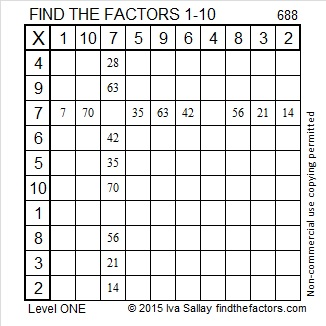# Why is 1503 a Friedman Number?

### Friedman Puzzle:

Can you find an expression equaling 1503 that uses 1, 5, 0, and 3 each exactly once, but in any order, and some combination of  +, -, ×, or ÷? For this particular Friedman puzzle, none of those digits are exponents. If you can solve this Friedman puzzle, you will know why 1503 is the 24th Friedman number. You can find the solution hidden someplace in this post. (By the way, another permutation of those digits, 1530, will be the 25th Friedman number!)

### Find the Factors Puzzle:

There are 14 clues in this level 2 puzzle. Use those clues and logic to place the factors 1 to 10 in both the first column and the top row. That’s how you start to turn this puzzle into a multiplication table!### Factors of 1503:

• 1503 is a composite number.
• Prime factorization: 1503 = 3 × 3 × 167, which can be written 1503 = 3² × 167.
• 1503 has at least one exponent greater than 1 in its prime factorization so √1503 can be simplified. Taking the factor pair from the factor pair table below with the largest square number factor, we get √1503 = (√9)(√167) = 3√167.
• The exponents in the prime factorization are 2 and 1. Adding one to each exponent and multiplying we get (2 + 1)(1 + 1) = 3 × 2 = 6. Therefore 1503 has exactly 6 factors.
• The factors of 1503 are outlined with their factor pair partners in the graphic below.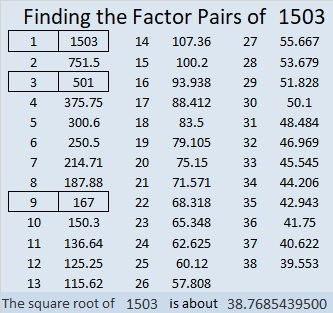Did you see the solution to the Friedman puzzle in that factor pair chart?

# 1435 is the 23rd Friedman Number

41 ×35 = 1435. Since the same digits are used on both sides of the equal sign that makes 1435 the 23rd Friedman number.

Since it’s good for puzzles to have a puzzle number to distinguish them from each other, I’ve given this one the number 1435. Can you solve it?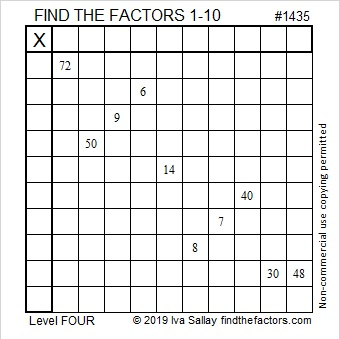Print the puzzles or type the solution in this excel file:  10 Factors 1432-1442

Here are a few more facts about the number 1435:

• 1435 is a composite number.
• Prime factorization: 1435 = 5 × 7 × 41
• 1435 has no exponents greater than 1 in its prime factorization, so √1435 cannot be simplified.
• The exponents in the prime factorization are 1, 1, and 1. Adding one to each exponent and multiplying we get (1 + 1)(1 + 1)(1 + 1) = 2 × 2 × 2 = 8. Therefore 1435 has exactly 8 factors.
• The factors of 1435 are outlined with their factor pair partners in the graphic below.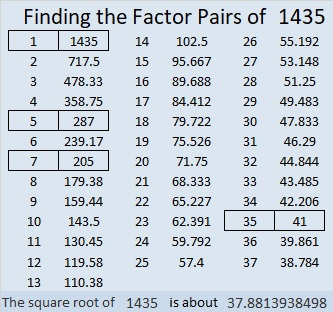1435 is also the hypotenuse of FOUR Pythagorean triples:
315-1400-1435 which is 35 times (9-40-41)
588-1309-1435 which is 7 times (84-187-205)
861-1148-1435 which is (3-4-5) times 287
931-1092-1435 which is 7 times (133-156-205)

# 1395 and Level 2

1391 is the 22nd Friedman number, and there are TWO reasons why!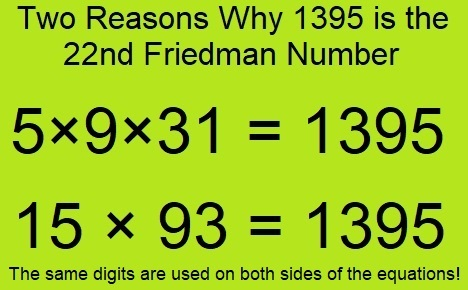See! Factoring numbers can be such an exciting adventure! Can you find the factors for this puzzle?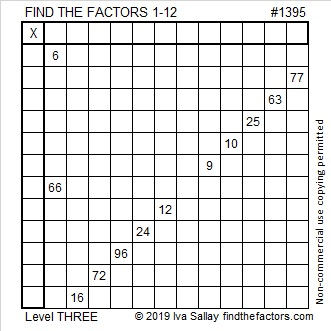Print the puzzles or type the solution in this excel file: 12 Factors 1389-1403\

Here’s more about the number 1395:

• 1395 is a composite number.
• Prime factorization: 1395 = 3 × 3 × 5 × 31, which can be written 1395 = 3² × 5 × 31
• 1395 has at least one exponent greater than 1 in its prime factorization so √1395 can be simplified. Taking the factor pair from the factor pair table below with the largest square number factor, we get √1395 = (√9)(√155) = 3√155
• The exponents in the prime factorization are 2, 1, and 1. Adding one to each exponent and multiplying we get (2 + 1)(1 + 1)(1 + 1) = 3 × 2 × 2 = 12. Therefore 1395 has exactly 12 factors.
• The factors of 1395 are outlined with their factor pair partners in the graphic below.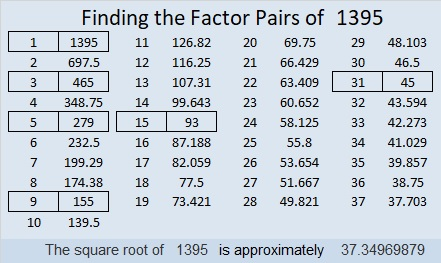You can see the reasons 1395 is the 22nd Friedman numbers in these factor pairs:
15 × 93 = 1395
45 × 31 = 5×9×31 = 1395, that one uses the digits in reverse order!

1395 is also the hypotenuse of a Pythagorean triple:
837-1116-1395 which is (3-4-5) times 279

# 1255 and Level 4

For this puzzle, you will have to study the twelve clues to figure out where to begin to find your first set of factors. You will then use those factors to figure out the next logical clue to use. Continue the process until all the factors are found. Good luck!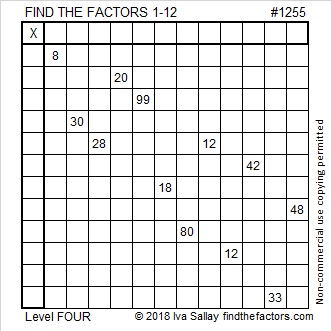Print the puzzles or type the solution in this excel file: 12 factors 1251-1258

Here is some information about the number 1255:

• 1255 is a composite number.
• Prime factorization: 1255 = 5 × 251
• The exponents in the prime factorization are 1 and 1. Adding one to each and multiplying we get (1 + 1)(1 + 1) = 2 × 2 = 4. Therefore 1255 has exactly 4 factors.
• Factors of 1255: 1, 5, 251, 1255
• Factor pairs: 1255 = 1 × 1255 or 5 × 251
• 1255 has no square factors that allow its square root to be simplified. √1255 ≈ 35.425981255 = 251 × 5
Check out those digits on both sides of the equation. Their sameness makes 1255 the18th Friedman number.

1255 is also the hypotenuse of a Pythagorean triple:
753-1004-1255 which is (3-4-5) times 251

# 1206 and Level 6

If you carefully study all the clues in this Level 6 puzzle and use logic, you should be able to solve the puzzle. Stick with it and you’ll succeed!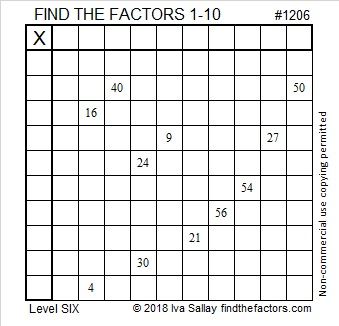Print the puzzles or type the solution in this excel file: 10-factors-1199-1210

Here are some facts about the number 1206:

• 1206 is a composite number.
• Prime factorization: 1206 = 2 × 3 × 3 × 67, which can be written 1206 = 2 × 3² × 67
• The exponents in the prime factorization are 1, 2, and 1. Adding one to each and multiplying we get (1 + 1)(2 + 1)(1 + 1) = 2 × 3 × 2 = 12. Therefore 1206 has exactly 12 factors.
• Factors of 1206: 1, 2, 3, 6, 9, 18, 67, 134, 201, 402, 603, 1206
• Factor pairs: 1206 = 1 × 1206, 2 × 603, 3 × 402, 6 × 201, 9 × 134, or 18 × 67,
• Taking the factor pair with the largest square number factor, we get √1206 = (√9)(√134) = 3√134 ≈ 34.72751Notice that 6·201 = 1206. Not very many numbers can equal themselves by using their own digits in a different way with +, -, ×, ÷, and/or parenthesis. That fact makes 1206 only the seventeenth Friedman Number.

1206 is also the sum of the twenty prime numbers from 19 to 103.

# 1022 Friedman Number Mystery

1022 is the 15th Friedman number. “What is a Friedman number and why is 1022 one of them?” you may ask. I will solve that little mystery for you. 1022 is a Friedman number because
2¹⁰ – 2 = 1022. Notice that the expression 2¹⁰ – 2 uses the digits 1, 0, 2, and 2 in some order and a subtraction sign. A Friedman number can be written as an expression that uses all of its own digits the exact number of times that they occur in the number. The expression must include at least one operator (+, -, ×, ÷) or a power. Parenthesis are allowed as long as the other rules are followed.

Now I would like you to solve the mystery of this puzzle using logic and the multiplication facts. Can you do it?Print the puzzles or type the solution in this excel file: 10-factors-1019-1027

1022 is the hypotenuse of a Pythagorean triple:
672-770-1022 which is 14 times (48-55-73)

• 1022 is a composite number.
• Prime factorization: 1022 = 2 × 7 × 73
• The exponents in the prime factorization are 1, 1, and 1. Adding one to each and multiplying we get (1 + 1)(1 + 1)(1 + 1) = 2 × 2 × 2 = 8. Therefore 1022 has exactly 8 factors.
• Factors of 1022: 1, 2, 7, 14, 73, 146, 511, 1022
• Factor pairs: 1022 = 1 × 1022, 2 × 511, 7 × 146, or 14 × 73
• 1022 has no square factors that allow its square root to be simplified. √1022 ≈ 31.96873# 688 is a Friedman number

688 is a palindrome in two different bases:

• 2002 in base 7; note that 2(343) + 0(49) + 0(7) + 2(1) = 688
• 494 in base 12; note that 4(144) + 9(12) + 4(1) = 688

688 is called a Friedman number because it can be expressed using only its own digits and +, -, x, ÷, ( ), and exponents. 688 = 8 x 86 so I made a factor tree based on that single multiplication fact:Since it’s such a fun number fact, I positioned it on top of today’s factoring puzzle, too.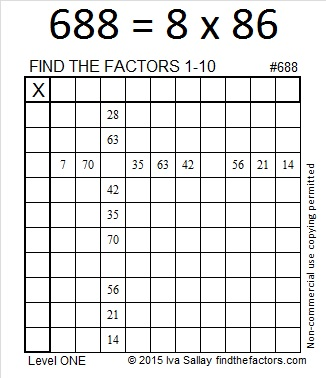Print the puzzles or type the solution on this excel file: 10 Factors 2015-11-23

—————————————————————————————————

• 688 is a composite number.
• Prime factorization: 688 = 2 x 2 x 2 x 2 x 43, which can be written 688 = 2⁴ x 43
• The exponents in the prime factorization are 4 and 1. Adding one to each and multiplying we get (4 + 1)(1 + 1) = 5 x 2 = 10. Therefore 688 has exactly 10 factors.
• Factors of 688: 1, 2, 4, 8, 16, 43, 86, 172, 344, 688
• Factor pairs: 688 = 1 x 688, 2 x 344, 4 x 172, 8 x 86, or 16 x 43
• Taking the factor pair with the largest square number factor, we get √688 = (√16)(√43) = 4√43 ≈ 26.229754.—————————————————————————————————